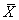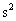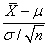Sections 5.3-5.5 Sampling Distributions and The Central Limit Theorem

Suppose a sample of n values is randomly selected from some population of values.  These n values are then averaged. The average of the n values is itself subject to randomness, since each of the values that make up the average is random. Suppose we sample n values from the population 100 times, each time averaging the n values to obtain the sample average. What is average value of these “averages”? What is the variability of these averages? What is their distribution?

We are interested in the sampling distribution of statistics, where a statistic is any quantity whose value can be calculated from sample data. Statistics can assume random values, thus they are random variables. For example, the sample average,,  is a statistic, as is, the sample variance. A random sample of size n is a collection of n independent random variables all having the same probability distribution.

We have the following results:

1. The average, or expected value, of the sample average is always equal to the expected value of the individual sample values.
2. If the samples are drawn from the population with replacement, the variability of the sample average is smaller than the variability of the individual averages, by a factor of 1/n.
3. If the sample size, n, is relatively large (n> 30) , the distribution of the sample average is approximately Normal, no matter what the distribution of the parent population. (If the parent population is a Normal distribution, the distribution of the averages is exactly Normal!)  Thus we have the following result, called the Central Limit Theorem.

When the sample size is large, the distribution ofis approximately the same as that of a standard Normal random variable.

Central Limit Theorem for Sample Proportions

Since the sample proportion of successful items in n trials, X/n, can be thought of as a average of n random variables, each taking value 0 or 1, then the sample proportion  has an approximate Normal distribution, with mean equal to the true proportion of successes in the population, p, and variability equal to p(1-p)/n. This approximation is good when if both np greater than or equal to ten and n(1-p) greater than or equal to ten.

The Distribution ofLinear Combinations

The mean of a linear combination of random variables is equal to the linear combination of the means of the individual random variables.

The variance of a linear combination of independent random variables is equal to the linear combination of the variances of the individual random variables, with scale factors in the linear combination squared.

The distribution of a linear combination of independent, Normally distributed random variables is also Normal. For example, if X1 and X2 are independent Normal r.v.s with means m1 and m2 and variances v1 and v2, respectively, then the distribution of X1-X2 is Normal with mean m1-m2 and variance v1+v2.